Open in App
Not now

# ∈-NFA of Regular Language L = 00(01+10)*11

• Difficulty Level : Basic
• Last Updated : 25 Mar, 2021

In Automata theory, Finite Automata can be classified into three types-

1. DFA
2. NFA
3. ∈-NFA

∈ means an empty input. That means the automaton can change its state even when it gets an empty symbol, i.e. ∈. That is what makes it different from NFA. Here is the formal definition of ∈-NFA.

```∈-NFA is defined in 5 tuple representation {Q, q0, Σ, δ, F} where
Q is the set of all states,
q0 is the initial state,
Σ is the set of input symbols,
δ is the transition function which is δ:Q × (Σ∪∈)⇢2Q and
F is the set of final states.```

There are a few special rules for the construction of ∈-NFA.

Simple rules for construction of ∈-NFA –

1. ∈-NFA for a+ :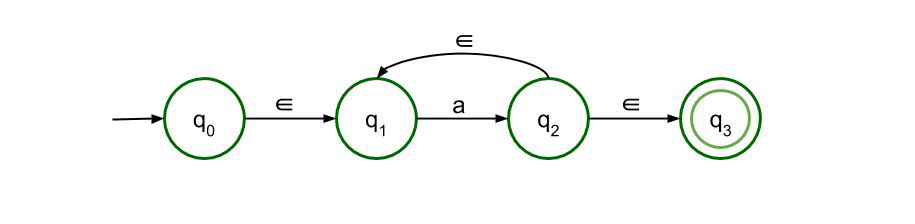‘a+’ represents that the expression must contain at least one a in order to be accepted by the automaton. The feedback connection from state q2 to state q1 is to denote that the expression can contain more than one a and it is denoted by ∈, so that two continuous ‘a’s can be accepted as the feedback need not be triggered by some other input.

2. ∈-NFA for a* :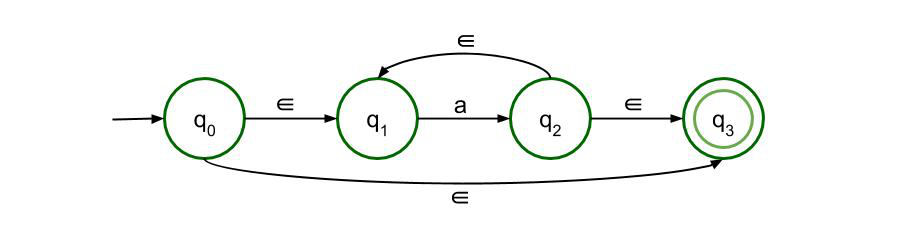‘a*’ represents that there can be zero or more ‘a’s in the expression. Like the previous one, there is feedback from state q2 to q1 but there is also a transition from state q0 to q3, i.e. straight from the initial state to the final state which shows that the expression will be accepted even if there is no ‘a’ in it.

3. ∈-NFA for a+b :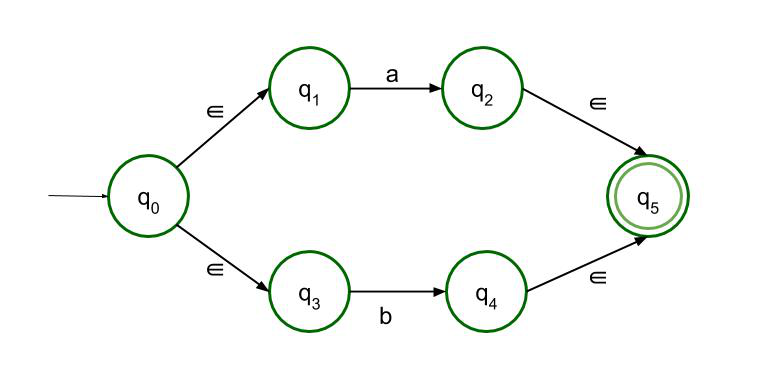‘a+b’ represents the OR logic. It means the expression can contain either a or b or both and it will be acceptable. So there are two paths, both of which lead to the final state.

4. ∈-NFA for ab :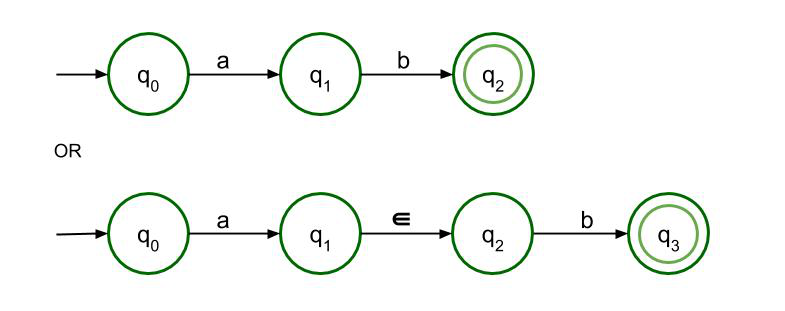‘ab’ represents the AND logic. It is also known as the concatenation rule. Here a must be followed by b strictly and only then will the expression be accepted. Both structures are allowed here but as it is ∈-NFA so the second structure is recommended.

∈-NFA for L = 00(01+10)*11 :

From the above-mentioned rules, ∈-NFA of Regular Language L = 00(01+10)*11 can be drawn.

It can be divided into three parts. The first part is ’00’, the second part is (01+10)* and the last part is ’11’. The first part and the third part are similar to the concatenation rule where both a and b denote the same symbol, either 0 or 1.

The second part is drawn using the fourth, third and second rule. We construct 01 and 10 using the fourth rule. Then we join them together using the third rule where a = 01 and b = 10. Then we apply the second rule and consider 01+10 as a unit. Here is the second part is drawn separately.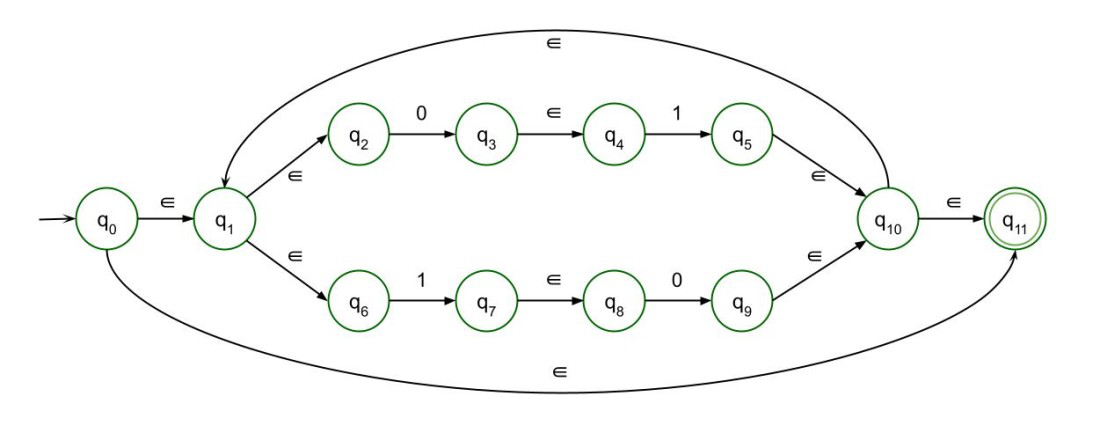The Final ∈-NFA will be :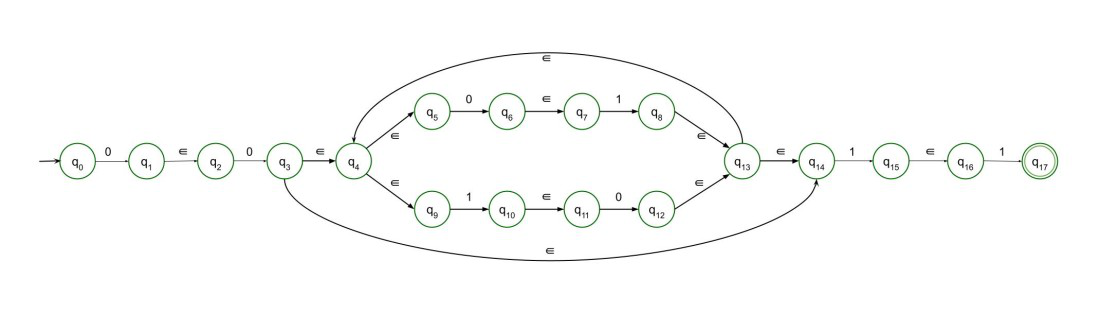My Personal Notes arrow_drop_up